# Algorithms for Dynamical Systems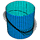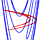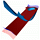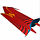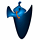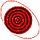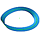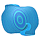Hinke's interest lies in the computation of invariant manifolds for dynamical systems. Most of this work is in collaboration with Bernd Krauskopf, University of Bristol. The main idea behind the algorithms is described in the paper Two-dimensional global unstable manifolds of maps (published electronically and in Int. J. Bifurcation & Chaos 8(3): 483-503, 1998).

#### Algorithms for mapsTwo special algorithms are derived from the paper Two-dimensional global unstable manifolds of maps. Software for one-dimensional global unstable manifolds of maps is available for use inside the DsTool environment; see the IMA-preprint and the paper for the IMA proceedings.The ideas for one-dimensional and two-dimensional manifolds are combined in a specialized algorithm for two-dimensional manifolds in quasiperiodically forced systems. The paper Growing 1D and quasi 2D unstable manifolds of maps describes both the one-dimensional and the quasi two-dimensional technique (published in J. Comput. Phys. 146(1): 404-419, 1998); see also the animations. The quasi 2D algorithm is used in the study of boundary crisis in quasiperiodically forced systems, research that is done together with Ulrike Feudel.

#### Algorithms for vector fieldsThe algorithm is also specialized for vector fields. The boundary of the computed part of the manifold is propagated further in steps that amount to solving a boundary value problem. Both unbounded manifolds and manifolds that converge to an attractor can be computed. Examples and animations are the two-dimensional stable manifold of the Lorenz system and the two-dimensional unstable manifold of Arneodo's system. This work will be published in Two-dimensional global manifolds of vector fields, CHAOS 9(3), 1999, with a multimedia supplement deposited in EPAPS.The above two examples are both three-dimensional vector fields. The computation of two-dimensional manifolds can straightforwardly be generalized to vector fields of higher dimension. The idea of growing stable and unstable manifolds using the formulation of a boundary value problem is generalizable to higher dimensional manifolds as well. However, the implementation and visualization of such a high dimensional object is quite complicated. More details are given in Global manifolds of vector fields: The general case.The same algorithm can be used for the computation of stable and unstable manifolds of periodic orbits. In this case, the manifold can be non-orientable and be topologically equivalent to a Möbius strip. How to adapt the algorithm to non-orientable manifolds is described in Non-orientable manifolds of periodic orbits.

Next: Dynamical Systems and Control